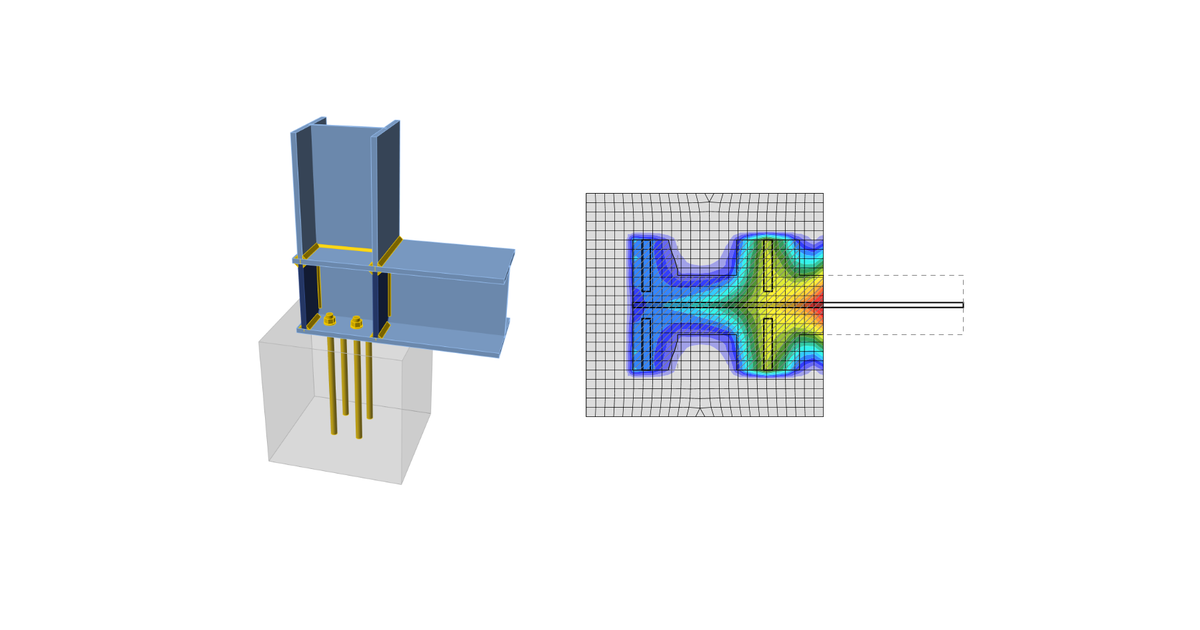### Choose language# Code-check of concrete block according to Chinese standard

$$The concrete below the base plate is simulated by Winkler subsoil with uniform stiffness, which provides the contact stresses. The average stress at the bearing area used for compressive check.Concrete in bearingUser can choose between local bearing capacity check of reinforced concrete pad (GB 50010, Equation 6.6.1-1) and plain concrete pad (GB 50010, Equation D.5.1-1).Reinforced concrete pad$F_l \le F_c = 1.35 \beta_c \beta_l f_c A_{ln}$Plain concrete pad$F_l \le F_c = \omega \beta_l f_{cc} A_l$where: Fl – compressive force Fc – compressive resistance βc – strength influential coefficient of concrete; βc = 1 for concrete grade up to C50, βc = 0.8 for concrete grade C80; linear interpolation is used for concrete grade between C50 and C80 $$\beta_l = \sqrt{\frac{A_b}{A_l}}$$ – concentration factor Ab – concrete supporting surface which is concentric to Al Al – base plate area in contact with concrete surface Aln – area Al with subtracted holes in base plate for anchors fc – design compressive strength of concrete; GB50010, Table 4.1.4-1 fcc = 0.85 fc – design compressive strength of plain concrete; GB50010, Table 4.1.4-1 ω – compression load distribution factor; ω = 0.75 for uneven load distribution, ω = 1.0 for even load distribution Transfer of shearThe shear action at the base plate is assumed to be transferred from the column to the concrete foundation by: Friction between base plate and concrete / grout Shear lug Anchor bolts AnchorsThe tensile forces in anchors include prying forces and are determined by finite element analysis.Anchors are not checked in the software.$$